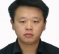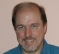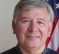## How to retrieve information from a short sequence of the sum of two cosine waves with close frequenciesStarted by 6 years ago9 replieslatest reply 6 years ago122 views

For example:

There is a short sequence with the time duration of 2 seconds. The sequence is the sum of two cosine waves with close frequencies, i.e. 0.11 Hz and 0.12 Hz. How can we retrieve the information of the amplitude, the frequency, and the phase of two cosine waves respectively?

Thanks!

[ - ]With difficulty and great imprecision.

Without sitting down and doing a bunch of math to back up my intuition, I would say that your best bet is going to be some sort of a nonliner optimization.  At worst, you'll need to optimize over both amplitudes, both phases, and some DC offset (because there's almost always some DC offset -- but if you're lucky you can rule that out).

Because the waves are so similar over such a short portion of their mutual cycle (if they're exactly 0.01Hz apart the cycle in question would be 100 seconds long, and you're only picking up on two seconds worth), there's not going to be much do distinguish them.  Not much to distinguish them means that the solver will, effectively, have high gains on the features that are different, and those high gains will amplify noise and distortion just as readily as they'll amplify real features.

I won't say that it's definitely a bad idea to even try, but you'll want to do some pretty careful sensitivity analysis before you start, to make sure you're not just wasting your time.

[ - ]Just to calibrate your thinking:  if the two sine waves are equal in magnitude, then their sum is equal to $$\cos \left(\omega_1 + \omega_2\right)t \cos \left( \omega_1 - \omega_2 \right) t$$, and $$\omega_1 - \omega_2$$ is equal to $$\frac{1}{100} \mathrm{Hz}$$.  So the envelope of the wave that you're trying to measure will advance through only 20 milliradians in the course of your measurement.  There's just not going to be a lot of information there to go on with.

[ - ]This is easy if there is no noise, and difficult to impossible in the presence of noise.  Your "difference frequency" is 0.01 Hz, which needs 100 seconds for a full "beating cycle" (so 50 seconds gets you a full pulse).  You have 2 seconds which is only 4% of the beat pulse, so this is why you are very sensitive to noise.  You don't even get a full cycle of the underlying sinusoids!

That said, in the noise-free case, it's straightforward.  You have six unknowns (amp, freq, and phase, for two sinusoids), so you need only six samples to set up six (nonlinear) equations in six unknowns.  In practice, there would normally be some fixed sampling rate fs > 8 1/3 Hz, and 2 seconds of that gets you 16 samples, which is sufficient for an overdetermined estimate of 6 parameters.  You can get some noise immunity by finding the six parameters yielding the best compromise fit across 16 samples.  You also get an idea how well it's going from the sum of squared errors you are minimizing (typically).  If the error over 16 samples is close to zero, then your signal model is probably valid.

Good luck!

[ - ]The case of two cosine waves is just an example, which simplifies the problem. A generic case is that the number of cosine waves is not known and it is in noisy environment. According to the resolution theory of Fourier transformation, the duration of the sequence should be longer than 100 seconds to distinguish a 0.01 Hz frequency difference. If we only have a short sequence with the time duration of 2 seconds, it is obviously impossible, through Fourier transformation, to distinguish the cosine waves whose frequency difference is as small as 0.01 Hz. Is there any possibility to distinguish these cosine waves in such a short sequence using other methods, such as super resolution methods?

[ - ]The easy way is to use a very high sample rate.

[ - ]From a philosophical point of view, you would need to resolve the two sinusoids in some context.  I don't think there's a way around that.  But I could be wrong.

Anyway, with a frequency difference of 0.01Hz, you would need a minimum of 100 seconds of data to resolve frequency to that resolution using normal DSP methods.

I never liked answers like this because they ignore practical things like noise.  One can work wonders with noiseless signals and mathematics!!  Nonetheless, consider an analytical solution:

s(t)=a0*cos(w0*t+p0)+a1*cos(w1*t+p1)

One could take 6 samples of the waveform to create 6 equations and, presumably solve for all the variables.  Well, as long as one takes into account the periodicity of phase and the cosines.  So the span of time covered in selecting the values of "t" should not be greater than the periodicity (if there is periodicity).  In the case you gave of 0.11Hz and 0.12Hz, the period would be 100 seconds.  Also, I should think that you would want to spread the sample points out because if they are too close together then numerical inaccuracies could be a problem.  It's an interesting challenge.

Actually, it appears that the situation as described is ill-conditioned.  Here's a plot of one period of the sum where the amplitudes are both 1.0, the phase difference is pi/2 and the frequencies are 0.11 and 0.12Hz.  The envelope has a period of 100 seconds as expected.I tried finding all the variables (amplitude, frequncy and phase) using Excel Solver to match a given set of the variables.  I used amplitudes both =1, 0.11Hz with phase 0 and 0.12Hz with phase of pi/2.

Even a very tiny change in phase of one of the sinusoids generates a significant difference in the error (between the "correct" sum and an "estimated" sum).  In general, the Excel Solver would not touch this problem .. even when the starting point was very close to the "solution".

Maybe there's a "trick" but I don't see it.

[ - ]You can separate two cosine waves if you will use parametric methods like Pisarenko method or MUSIC.

[ - ]